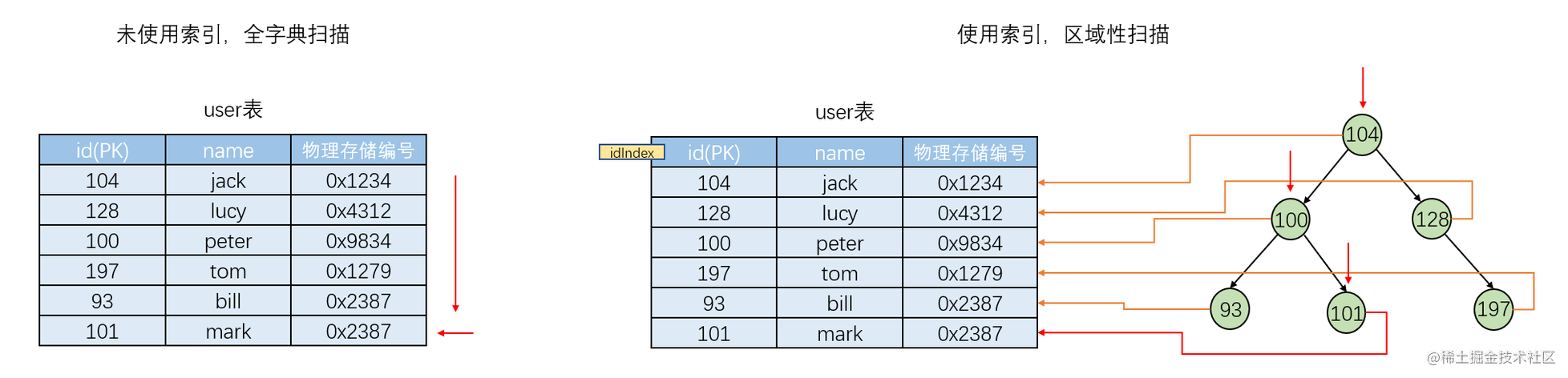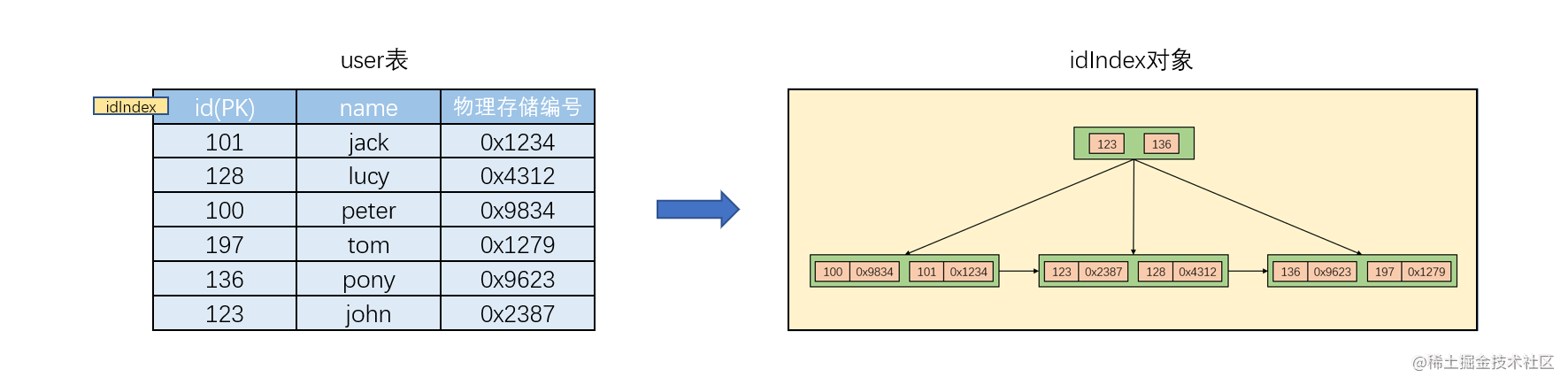# 【MySQL】基础部分(事务，索引)

## 事务

### 1. 概述

1. 将A账户的钱减去10000。
2. 将B账户的钱增加10000。

### 2. 实现

#### 2.1 提交事务

``````commit;

#### 2.2 回滚事务

``````rollback;

#### 2.3 MySQL自动提交机制

MySQL默认情况下支持事务自动提交，就是默认将每一条DML都看作一个事务，每一条DML执行完毕后就自动提交事务。

``````start transaction;

### 3. 特性

• 原子性（A—Atomicity）：事务是最小的工作单元，不可再分。在同一个事务中，所有操作必须同时成功，或者同时失败，不会结束在中间某个环节。如果事务在执行过程中发生错误，会被恢复（Rollback）到事务开始前的状态，就像这个事务从来没有执行过一样。
• 一致性（C—Consistency）：在事务开始之前和事务结束以后，数据库的完整性没有被破坏。一致性可以理解为使用事务的目的，而原子性、隔离性、持久性均是保证一致性的手段，需要通过应用程序代码来保证一致性。
• 隔离性（I—Isolation）：在事务并发执行时，事务和事务之间有一定的隔离，事务内部的操作不能互相干扰。如果多个事务可以同时操作一个数据，那么就会产生脏读、不可重复读、幻读的问题。
• 持久性（D—Durability）：事务最终结束的一个保障。事务提交，就相当于将没有持久化的数据持久化到硬盘上。

### 4. 事务隔离级别

#### 4.1 读未提交

T1begin;begin;
T2select * from user where id = 3;

T3select * from user where id = 3;

T4update user set age = 18 where id = 3;
T5select * from user where id = 3;

T6commit;
T7select * from user where id = 3;

T8commit;
T9select * from user where id = 3;

T1begin;begin;
T2select * from user where id = 3;

T3select * from user where id = 3;

T4update user set age = 20 where id = 3;
T5select * from user where id = 3;

T6rollback;
T7update user set age = age + 1 where id = 3;
T8select * from user where id = 3;

T9commit;
T10select * from user where id = 3;

#### 4.2 读已提交

T1begin;begin;
T2select * from user where id = 3;

T3select * from user where id = 3;

T4update user set age = 18 where id = 3;
T5select * from user where id = 3;

T6commit;
T7select * from user where id = 3;

T8commit;
T9select * from user where id = 3;

Oracle默认的数据库隔离级别是读已提交。

#### 4.3 可重复读

T1begin;begin;
T2select * from user where id = 3;

T3select * from user where id = 3;

T4update user set age = 18 where id = 3;
T5select * from user where id = 3;

T6commit;
T7select * from user where id = 3;

T8commit;
T9select * from user where id = 3;

T1begin;begin;
T2select * from user where age = 20；

T3insert into user values (10，george，20);
T4commit;
T5select * from user where age = 20；

T6update user set name = 'peter' where age = 20;

T7select * from user where age = 20；

T8commit;

MySQL默认的数据库隔离级别就是可重复读。

#### 4.5 MySQL设置隔离级别

``````select @@tx_isolation;

``````set global transaction isolation level read uncommited;

``````set global transaction isolation level read commited;

``````set global transaction isolation level repeatable read;

``````set global transaction isolation level serializable;

### 5. 更新丢失

#### 5.1 第一类更新丢失

SQL92没有定义这种现象，标准定义的所有隔离级别都不允许第一类丢失更新发生。基本上数据库的使用者不需要关心此类问题。

T1begin;begin;
T2select * from user where id = 3;

T3select * from user where id = 3;

T4update user set age = age + 1 where id = 3;
T5select * from user where id = 3;

T6update user set age = age - 1 where id = 3;
T7select * from user where id = 3;

T8commit;
T9rollback;
T10select * from user where id = 3;

#### 5.2 第二类更新丢失

T1begin;begin;
T2select * from user where id = 3;

T3select * from user where id = 3;

T4update user set age = age + 1 where id = 3;
T5select * from user where id = 3;

T6update user set age = age - 1 where id = 3;
T7select * from user where id = 3;

T8commit;
T9commit;
T10select * from user where id = 3;

## 索引

### 1. 概述

MySQL有两种查询方式：

• 全字典扫描：从表的第一行开始，逐行进行扫描匹配，效率相对较慢。
• 区域性扫描：先通过查看表的索引确定一个大概搜索范围，然后从该范围的第一行开始，逐行进行扫描匹配，效率相对较高。### 2. 存储位置

• InnoDB：索引存储在一个逻辑名称为tablespace的空间中。
• MyISAM：索引存储在一个 `.MYI` 文件中。
• MEMORY：索引存储在内存中。

### 3. 分类

• 单值索引：一个索引只包含单个列。
• 复合索引：一个索引包含多个列。

### 4. 优劣势

• 类似于书籍目录，提高数据检索效率，降低数据库IO成本。
• 通过索引对数据进行排序，降低数据排序成本，降低CPU消耗。

• 实际上索引也是一张表，该表中保存了主键、索引字段和指向表中哪一行的记录，因此索引是要占一定空间的。
• 索引降低了更新表的速度。因为在对表进行insert、delete和update时，MySQL不仅要更新数据，还要更新索引信息。

### 5. 实现原理

• 被添加索引的字段的每一行的内容。
• 每一行数据对应的物理存储编号。

``````select * from xxx where 物理编号 = xxx;### 6. 添加条件

• 数据量庞大，由于硬件环境不同，需要进行测试。
• 该字段的数据经常被扫描，也就是说该字段经常出现where或者having的后面作为查询的条件。
• 该字段很少会被DML操作（insert，delete，update），因为如果该字段被DML操作后，索引会重新组织排序。

### 7. 创建和删除

``````create index 表名_字段名_index on 表名(字段名);

``````create index 表名_字段名1_字段名2_index on 表名(字段名1, 字段名2);

``````drop index 表名_字段名_index on 表名;

### 8. 失效

#### 8.1 检测失效

MySQL中如何查看一条DQL是否使用索引进行检索：

``````explain select语句

#### 8.2 失效情况一

``````select * from emp where ename like '%T';

#### 8.5 失效情况四

``````select * from emp where sal + 1 = 800;

#### 8.6 失效情况五

``````select * from emp where lower(name) = 'smith';